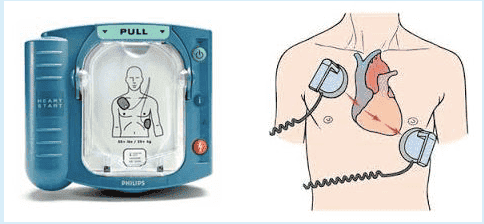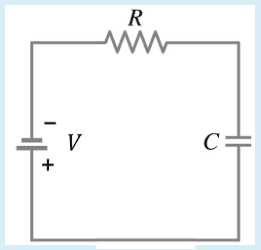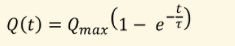# Charge Stored on a Capacitor

Jess_18033152

## Homework Statement

Defibrillation[/B] is a common treatment for life-threatening cardiac arrhythmias and ventricular fibrillation. The defibrillator applies a current across the heart (shown by the red arrows in the image below) for a short time (a few milliseconds), disrupting the arrhythmia and hopefully allowing the normal heartbeat to resume. The electric current is supplied by a device called a defibrillator (shown on the left in the image below).The defibrillator uses a capacitor to store the charge that will be delivered in the current. The capacitor forms part of an RC-circuit, as shown in the diagram below:Calculate the amount of charge stored on the capacitor after the capacitor has been charging for 1.10 s.

- The capacitance of the capacitor is 28.1 μF and the resistance of the resistor is 20.4 kΩ.
- Time constant of the RC circuit within the defibrillator = 0.573 s
- The amount of charge stored on the capacitor when the capacitor is fully charged to 2.75 kV = 0.0773 C

## Homework Equations## The Attempt at a Solution

C = T/R
= 1.10/20400
= 5.39x10^-5 F was not correct

Not sure where to go for this question as I don't understand the following equation;#### Attachments

Mentor
Your images are not visible. Please use the UPLOAD button on the editor panel to upload your images to our servers; in many cases, off-site images on image-storage sites are not publicly available.

•berkeman
Mentor
C = T/R
= 1.10/20400
= 5.39x10^-5 F was not correct
Note that in the equation T = RC:
C is the capacitance, which is given and fixed, not charge.
T is the time constant for the RC circuit, another constant that is given.

The time constant is used to describe the charging and discharging of a capacitor as an exponential function of time. (Look it up!)